# Class 6 Maths NCERT Solutions for Chapter 7 Fractions Ex – 7.1

## Fractions

Question 1.
Write the fraction representing the shaded portion.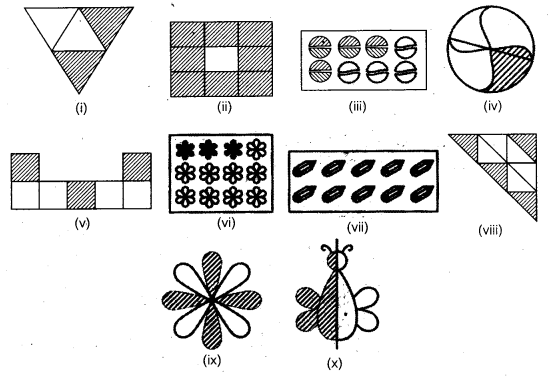Solution:
The fraction representing the shaded portion in the given diagram are as under: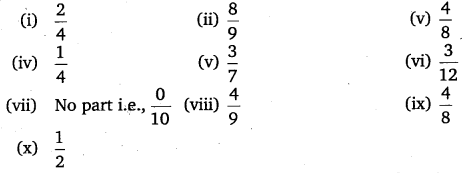Question 2.
Colour the part according to the given fraction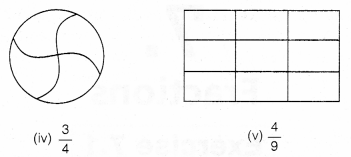Solution:
The figures are shaded as per indicated fraction shown against them.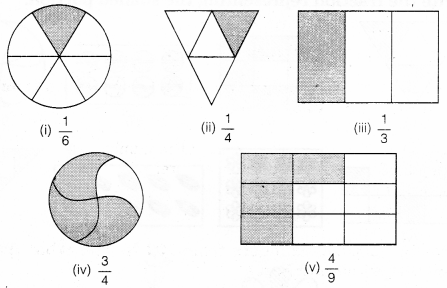Question 3.
Identify the error, if any?Solution:
In all the figures, the shaded portions do not represent the given fractions.

Question 4.
What fraction of a day is 8 hours?

Solution:
Since, there are 24 hours in a day, therefore, the required fraction$=\frac { 8 }{ 24 } =\frac { 1 }{ 3 }$.

Question 5.
What fraction of an hour is 40 minutes?

Solution:
Since, there are 60 minutes in an hour, therefore, the required fraction$=\frac { 40 }{ 60 } =\frac { 2 }{ 3 }$.

Question 6.
Arya, Abhimanyu and Vivek shared lunch. Arya has brought two sandwiches, one made of vegetable and one of jam. The other two boys forgot to bring their lunch. Arya agreed to share his sandwiches so that each person will have an equal share of each sandwich.

(a) How can Arya divide his sandwiches so that each person has an equal share?
(b) What part of a sandwich will each boy receive?

Solution:
(a)
To divide 2 sandwiches brought by Arya equally among Arya, Abhimanyu and Vivek (i.e., 3 persons), we have to divide each sandwich into 3 equal parts. Then two parts thus obtained be given to each of them.
(b)$\frac { 1 }{ 3 }$ of a sandwich will be received by each boy but in total each will get$\frac { 2 }{ 3 }$.

Question 7.
Kanchan dyes dresses. She had to dye 30 dresses. She has so far finished 20 dresses. What fraction of dresses has she finished?

Solution:
Total number of dresses = 30
Work finished = 20
∴ The fraction of dresses she has finished =$=\frac { 20 }{ 30 } =\frac { 2 }{ 3 }$.

Question 8.
Write the natural numbers from 2 to 12. What fraction of them are prime numbers?

Solution:
Natural numbers from 2 to 12 are:
2, 3, 4, 5, 6, 7, 8, 9, 10, 11, 12 i.e., 11 in number.
Prime numbers out of these numbers are:
2, 3, 5, 7, 11 i.e., 5 in number.
∴ Required fraction =$=\frac { 4 }{ 12 } =\frac { 1 }{ 3 }$

Question 9.
Write the natural numbers from 102 to 113. What fraction of them are prime numbers?

Solution:
Natural numbers from 102 to 113 are:
102, 103, 104, 105, 106, 107, 108, 109, 110, 111, 112, 113 i.e., 12 in number.
Prime numbers out of the above numbers are:
103, 107, 109, 113 i.e., 4 in number.
∴ Required fraction =$=\frac { 4 }{ 12 } =\frac { 1 }{ 3 }$

Question 10.
What fraction of these circles have X’s in them?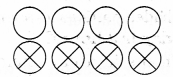Solution:
∴Required fraction =$=\frac { 4 }{ 8 } =\frac { 1 }{ 2 }$

Question 11.
Kristin received a CD player for her birthday. She bought 3 CDs and received 5 others as gifts. What fraction of her total CDs did she buy and what fraction did she receive as gifts?

Solution:
Total number of CDs = 3 + 5 = 8
Number of CDs purchased = 3
Fraction of CDs purchased =$\frac { 3 }{ 8 }$
Fraction of CDs received as gift =$\frac { 5 }{ 8 }$ .

#### Class 6 Maths NCERT Solutions for Chapter 7 Fractions Ex – 7.2

Lorem ipsum dolor sit amet, consectetur adipiscing elit. Phasellus cursus rutrum est nec suscipit. Ut et ultrices nisi. Vivamus id nisl ligula. Nulla sed iaculis ipsum.

Company Name